Do you like the article?
Share it with others -

Use new possibilities of MetaTrader 5

# Modeling time series using custom symbols according to specified distribution laws

30 October 2018, 11:49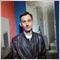4
9 346

### Introduction

The MetaTrader 5 trading terminal allows creating and using custom symbols in work. Traders have the ability to test their own currency pairs and other financial instruments. The article proposes ways of creating and removing custom symbols, generation of ticks and bars according to the specified distribution laws.

It also proposes methods for simulating the trend and various chart patterns. Proposed ready-made scripts for working with custom symbols with minimal settings allow traders who do not have MQL5 programming skills to use the full potential of custom symbols.

### Creating and removing custom symbols

This earlier article presents a way of creating custom symbols in the "Symbols" window in MetaTrader 5 based on existing symbols.

We suggest automation of this process using a simple setting with minimal configuration.

The script has four input parameters:

• name of the custom symbol,
• short name of the currency pair or financial instrument,
• full name of the currency pair or financial instrument,
• short name of the base currency or financial instrument, if the symbol is created based on the base symbol,
Here is the script's code (the script is located in the CreateSymbol.mq5 file attached to the article):

```//+------------------------------------------------------------------+
//|                                                 CreateSymbol.mq5 |
//|                                                  Aleksey Zinovik |
//|                                                                  |
//+------------------------------------------------------------------+
#property script_show_inputs
#property version   "1.00"
//+------------------------------------------------------------------+
//| Script program start function                                    |
//+------------------------------------------------------------------+
input string SName="ExampleCurrency";
input string CurrencyName="UCR";
input string CurrencyFullName="UserCurrency";
input string BaseName="EURUSD";
//+------------------------------------------------------------------+
//|                                                                  |
//+------------------------------------------------------------------+
void OnStart()
{
ResetLastError();
// create symbol
if(!CustomSymbolCreate(SName,"\\Forex"))
{
if(SymbolInfoInteger(SName,SYMBOL_CUSTOM))
else
Print("Error creating symbol. Error code: ",GetLastError());
}
else
{
if(BaseName=="")// create new
{
// String type properties
if((SetProperty(SName,SYMBOL_CURRENCY_BASE,CurrencyName,"")) && // base currency
(SetProperty(SName,SYMBOL_CURRENCY_PROFIT,"USD",""))&&                         // profit currency
(SetProperty(SName,SYMBOL_CURRENCY_MARGIN,"USD",""))&&                         // margin currency
(SetProperty(SName,SYMBOL_DESCRIPTION,CurrencyName,""))&&                      // the string description of the symbol (full name)
(SetProperty(SName,SYMBOL_BASIS,"","")) &&                                     // the name of the underlaying asset for the custom symbol
(SetProperty(SName,SYMBOL_FORMULA,"","")) &&                                   // the formula used for custom symbol pricing
(SetProperty(SName,SYMBOL_ISIN,"","")) &&                                      // the name of a trading symbol in the ISIN system
(SetProperty(SName,SYMBOL_PAGE,"","")) &&                                      // the web page containing symbol information
// Integer type properties
(SetProperty(SName,SYMBOL_CHART_MODE,SYMBOL_CHART_MODE_BID,"")) &&             // plotting charts by Bid prices
(SetProperty(SName,SYMBOL_DIGITS,5,"")) &&                                     // precision
(SetProperty(SName,SYMBOL_TICKS_BOOKDEPTH,10,"")) &&                           // depth of the order book
(SetProperty(SName,SYMBOL_BACKGROUND_COLOR,White,""))&&                        // the color of the background used for the symbol in Market Watch
(SetProperty(SName,SYMBOL_ORDER_GTC_MODE,SYMBOL_ORDERS_GTC,""))&&              // expiration of StopLoss and TakeProfit of orders: good until canceled
(SetProperty(SName,SYMBOL_FILLING_MODE,SYMBOL_FILLING_FOK,""))&&               // order execution mode: fill or kill
(SetProperty(SName,SYMBOL_EXPIRATION_MODE,SYMBOL_EXPIRATION_GTC,""))&&         // order expiration mode: unlimited in time, until canceled explicitly
(SetProperty(SName,SYMBOL_ORDER_MODE,127,"")) &&                               // order types: all order types
(SetProperty(SName,SYMBOL_TRADE_CALC_MODE,SYMBOL_CALC_MODE_FOREX,""))&&        // method of calculating the contract value
(SetProperty(SName,SYMBOL_MARGIN_HEDGED_USE_LEG,false,""))&&                   // calculation of the hedged margin using the larger leg
(SetProperty(SName,SYMBOL_SWAP_MODE,SYMBOL_SWAP_MODE_POINTS,""))&&             // swap calculation model: calculation of swap in points
(SetProperty(SName,SYMBOL_SWAP_ROLLOVER3DAYS,WEDNESDAY,"")) &&                 // triple-swap day
(SetProperty(SName,SYMBOL_OPTION_MODE,0,"")) &&                                // option type
(SetProperty(SName,SYMBOL_OPTION_RIGHT,0,"")) &&                               // option right
(SetProperty(SName,SYMBOL_TRADE_STOPS_LEVEL,0,"")) &&                          // minimum distance in points from the current close price for setting Stop orders
(SetProperty(SName,SYMBOL_START_TIME,0,"")) &&                                 // symbol trading start date (usually used for futures)
(SetProperty(SName,SYMBOL_EXPIRATION_TIME,0,"")) &&                            // symbol trading end date (usually used for futures)
// Double type properties
(SetProperty(SName,SYMBOL_OPTION_STRIKE,0,"")) &&                              // option strike price
(SetProperty(SName,SYMBOL_SESSION_PRICE_LIMIT_MAX,0,"")) &&                    // the minimum allowable price value for the session
(SetProperty(SName,SYMBOL_SESSION_PRICE_LIMIT_MIN,0,"")) &&                    // the maximum allowable price value for the session
(SetProperty(SName,SYMBOL_SESSION_PRICE_SETTLEMENT,0,"")) &&                   // the settlement price of the current session
(SetProperty(SName,SYMBOL_TRADE_ACCRUED_INTEREST,0,"")) &&                     // accrued interest (for bonds)
(SetProperty(SName,SYMBOL_TRADE_FACE_VALUE,0,"")) &&                           // face value (for bonds)
(SetProperty(SName,SYMBOL_TRADE_LIQUIDITY_RATE,0,"")) &&                       // liquidity rate(used for collateral symbols)
(SetProperty(SName,SYMBOL_TRADE_TICK_SIZE,0.00001,"")) &&                      // the minimum price change
(SetProperty(SName,SYMBOL_POINT,0.00001,"")) &&                                // point value
(SetProperty(SName,SYMBOL_VOLUME_MIN,0.01,"")) &&                              // the minimum volume for deal execution
(SetProperty(SName,SYMBOL_VOLUME_MAX,500.00,"")) &&                            // the maximum volume for deal execution
(SetProperty(SName,SYMBOL_VOLUME_STEP,0.01,"")) &&                             // the minimum volume change step for deal execution
(SetProperty(SName,SYMBOL_VOLUME_LIMIT,0,"")) &&                               // the maximum allowed total volume of an open position and pending orders in one direction (either buy or sell) for this symbol
(SetProperty(SName,SYMBOL_MARGIN_INITIAL,0,"")) &&                             // initial margin
(SetProperty(SName,SYMBOL_MARGIN_MAINTENANCE,0,"")) &&                         // maintenance margin
(SetProperty(SName,SYMBOL_MARGIN_HEDGED,100000,"")) &&                         // size of a contract or margin for one lot of oppositely directed positions for one symbol
(SetProperty(SName,SYMBOL_SWAP_LONG,-0.7,"")) &&                               // Long swap value
(SetProperty(SName,SYMBOL_SWAP_SHORT,-1,"")))                                  // Short swap value
Print("Symbol ",SName," created successfully");
else
Print("Error setting symbol properties. Error code: ",GetLastError());
}
else// create based on the base symbol
{
if((SetProperty(SName,SYMBOL_CURRENCY_BASE,CurrencyName,"")) &&
(SetProperty(SName,SYMBOL_CURRENCY_PROFIT,"",BaseName)) &&
(SetProperty(SName,SYMBOL_CURRENCY_MARGIN,"",BaseName)) &&
(SetProperty(SName,SYMBOL_DESCRIPTION,CurrencyFullName,"")) &&
(SetProperty(SName,SYMBOL_BASIS,"",BaseName)) &&
(SetProperty(SName,SYMBOL_FORMULA,"",BaseName)) &&
(SetProperty(SName,SYMBOL_ISIN,"",BaseName)) &&
(SetProperty(SName,SYMBOL_PAGE,"",BaseName)) &&

(SetProperty(SName,SYMBOL_CHART_MODE,0,BaseName)) &&
(SetProperty(SName,SYMBOL_DIGITS,0,BaseName)) &&
(SetProperty(SName,SYMBOL_TICKS_BOOKDEPTH,0,BaseName)) &&
(SetProperty(SName,SYMBOL_BACKGROUND_COLOR,0,BaseName)) &&
(SetProperty(SName,SYMBOL_ORDER_GTC_MODE,0,BaseName)) &&
(SetProperty(SName,SYMBOL_FILLING_MODE,0,BaseName)) &&
(SetProperty(SName,SYMBOL_EXPIRATION_MODE,0,BaseName)) &&
(SetProperty(SName,SYMBOL_ORDER_MODE,0,BaseName)) &&
(SetProperty(SName,SYMBOL_MARGIN_HEDGED_USE_LEG,0,BaseName)) &&
(SetProperty(SName,SYMBOL_SWAP_MODE,0,BaseName)) &&
(SetProperty(SName,SYMBOL_SWAP_ROLLOVER3DAYS,0,BaseName)) &&
(SetProperty(SName,SYMBOL_OPTION_MODE,0,BaseName)) &&
(SetProperty(SName,SYMBOL_OPTION_RIGHT,0,BaseName)) &&
(SetProperty(SName,SYMBOL_START_TIME,0,BaseName)) &&
(SetProperty(SName,SYMBOL_EXPIRATION_TIME,0,BaseName)) &&

(SetProperty(SName,SYMBOL_OPTION_STRIKE,0,BaseName)) &&
(SetProperty(SName,SYMBOL_SESSION_PRICE_LIMIT_MAX,0,BaseName)) &&
(SetProperty(SName,SYMBOL_SESSION_PRICE_LIMIT_MIN,0,BaseName)) &&
(SetProperty(SName,SYMBOL_SESSION_PRICE_SETTLEMENT,0,BaseName)) &&
(SetProperty(SName,SYMBOL_POINT,0,BaseName)) &&
(SetProperty(SName,SYMBOL_VOLUME_MIN,0,BaseName)) &&
(SetProperty(SName,SYMBOL_VOLUME_MAX,0,BaseName)) &&
(SetProperty(SName,SYMBOL_VOLUME_STEP,0,BaseName)) &&
(SetProperty(SName,SYMBOL_VOLUME_LIMIT,0,BaseName)) &&
(SetProperty(SName,SYMBOL_MARGIN_INITIAL,0,BaseName)) &&
(SetProperty(SName,SYMBOL_MARGIN_MAINTENANCE,0,BaseName)) &&
(SetProperty(SName,SYMBOL_MARGIN_HEDGED,0,BaseName)) &&
(SetProperty(SName,SYMBOL_SWAP_LONG,0,BaseName)) &&
(SetProperty(SName,SYMBOL_SWAP_SHORT,0,BaseName)))
Print("Symbol ",SName," created successfully");
else
Print("Error setting symbol properties. Error code: ",GetLastError());
}
if(SymbolSelect(SName,true))
Print("Symbol ",SName," selected in Market Watch");
else
Print("Error selecting symbol in Market Watch. Error code: ",GetLastError());
}
}
// function for setting the symbol properties
bool SetProperty(string SymName,ENUM_SYMBOL_INFO_STRING SProp,string PropValue,string BaseSymName)
{
ResetLastError();
if(BaseSymName=="")
{
if(CustomSymbolSetString(SymName,SProp,PropValue))
return true;
else
Print("Error setting symbol property: ",SProp,". Error code: ",GetLastError());
}
else
{
string SValue=SymbolInfoString(BaseSymName,SProp);
if(CustomSymbolSetString(SymName,SProp,SValue))
return true;
else
Print("Error setting symbol property: ",SProp,". Error code: ",GetLastError());
}
return false;
}
//+------------------------------------------------------------------+
//|                                                                  |
//+------------------------------------------------------------------+
bool SetProperty(string SymName,ENUM_SYMBOL_INFO_INTEGER IProp,long PropValue,string BaseSymName)
{
ResetLastError();
if(BaseSymName=="")
{
if(CustomSymbolSetInteger(SymName,IProp,PropValue))
return true;
else
Print("Error setting symbol property: ",IProp,". Error code: ",GetLastError());
}
else
{
long IValue=SymbolInfoInteger(BaseSymName,IProp);
if(CustomSymbolSetInteger(SymName,IProp,IValue))
return true;
else
Print("Error setting symbol property: ",IProp,". Error code: ",GetLastError());
}
return false;
}
//+------------------------------------------------------------------+
//|                                                                  |
//+------------------------------------------------------------------+
bool SetProperty(string SymName,ENUM_SYMBOL_INFO_DOUBLE DProp,double PropValue,string BaseSymName)
{
ResetLastError();
if(BaseSymName=="")
{
if(CustomSymbolSetDouble(SymName,DProp,PropValue))
return true;
else
Print("Error setting symbol property: ",DProp,". Error code: ",GetLastError());
}
else
{
double DValue=SymbolInfoDouble(BaseSymName,DProp);
if(CustomSymbolSetDouble(SymName,DProp,DValue))
return true;
else
Print("Error setting symbol property: ",DProp,". Error code: ",GetLastError());
}
return false;
}
//+------------------------------------------------------------------+
```

Let us consider the script's code in more detail. First, an attempt is made to create a symbol using the CustomSymbolCreate function:

```if(!CustomSymbolCreate(SName,"\\Forex"))
{
if(SymbolInfoInteger(SName,SYMBOL_CUSTOM))
else
Print("Error creating symbol. Error code: ",GetLastError());
}
```

The symbol is created in the Custom/Forex folder. If you want to create a custom subfolder (custom group) in the folder Custom, specify its name in the second parameter of the CustomSymbolCreate function.

Next the properties of the created symbol are set. If the BaseName parameter is not defined, user-defined parameters of the symbol are set. For example, the properties of the EURUSD currency pair are listed:

```    // String type properties
if((SetProperty(SName,SYMBOL_CURRENCY_BASE,CurrencyName,"")) && // base currency
(SetProperty(SName,SYMBOL_CURRENCY_PROFIT,"USD",""))&&                         // profit currency
(SetProperty(SName,SYMBOL_CURRENCY_MARGIN,"UCR",""))&&                         // margin currency
...
```

For convenience, the properties are divided into groups. First, the properties of the type String are set, then Integer, and then Double. In case these properties are set successfully, a message about successful creation of a symbol is written to the log. Otherwise, the code of the error that occurred when setting the symbol properties is written to the log.

If the value of the BaseName parameter is not empty, the symbol properties are copied from the properties of the base symbol, the name of which is defined by the BaseName parameter. For example, it can be EURUSD, USDCAD, GBPUSD and others.

Properties of the symbol are set by the SetProperty function, which is described after the code of the main script function. This function is not used in other scripts; therefore, it is not moved to a separated included class.

For properties of type String, Integer and Double, separate instances of the SetProperty function are created. The functions CustomSymbolSetString, CustomSymbolSetInteger, CustomSymbolSetDouble are used for setting the properties of the custom symbol. The functions SymbolInfoString, SymbolInfoInteger, SymbolInfoDouble are used to get the properties of the base symbol.

After successfully setting the properties of the created custom symbol, it is selected in the Market Watch using the SymbolSelect function:

```  if(SymbolSelect(SName,true))
Print("Symbol ",SName," selected in Market Watch");
else
Print("Error selecting symbol in Market Watch. Error code: ",GetLastError());
```

To open the chart of the created symbol, it is necessary to load ticks or bars into the symbol. The script for generating ticks and bars will be discussed below.

Now consider the process of deleting a custom symbol. If you want to delete a custom symbol by selecting it on the Symbols tab, you will not always be able to do this: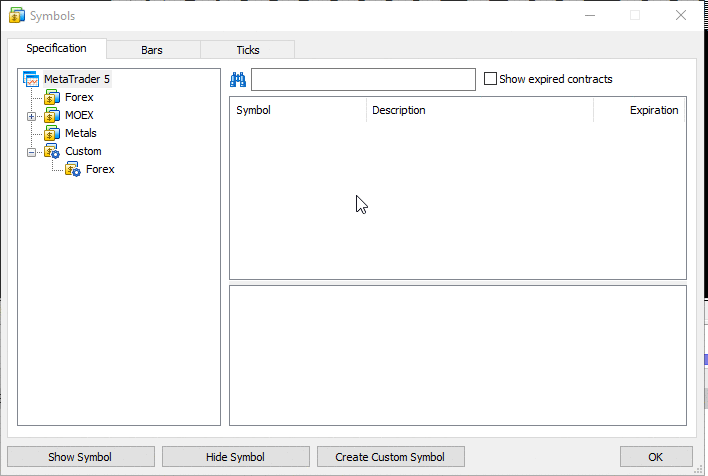Fig. 1. Attempt to delete a symbol that is selected in the Market Watch

In order to delete a symbol, it must be removed from the Market Watch — this can be done by double-clicking the symbol in the Symbols window. At the same time, there should be no open charts and positions for the symbol you want to delete. Therefore, it is necessary to close all charts and positions on this symbol manually. This is not a fast process, especially if many charts are open for this symbol. Here is an example of symbol deletion implemented as a small script (the script is attached to the article in the DeleteSymbol.mq5 file):

```//+------------------------------------------------------------------+
//|                                                 DeleteSymbol.mq5 |
//|                                                  Aleksey Zinovik |
//|                                                                  |
//+------------------------------------------------------------------+
#property script_show_inputs
#property version   "1.00"
//+------------------------------------------------------------------+
//| Script program start function                                    |
//+------------------------------------------------------------------+
input string SName="ExampleCurrency";
//+------------------------------------------------------------------+
//| Script program start function                                    |
//+------------------------------------------------------------------+
void OnStart()
{
ResetLastError();
if(SymbolInfoInteger(SName,SYMBOL_CUSTOM))// if the symbol exists
{
if(!CustomSymbolDelete(SName))// attempt to delete
{
if(SymbolInfoInteger(SName,SYMBOL_SELECT))// if it is selected in the Market Watch
{
if(SymbolSelect(SName,false))// attempt to disable and delete
{
if(!CustomSymbolDelete(SName))
Print("Error deleting symbol ",SName,". Error code: ",GetLastError());
else
Print("Symbol ",SName," deleted successfully");
}
else
{
// attempt to close charts with the symbol
int i=0;
long CurrChart=ChartFirst();
int i_id=0;
long ChartIDArray[];
while(CurrChart!=-1)
{
// loop through the list of charts and store identifiers to open charts of the symbol SName
if(ChartSymbol(CurrChart)==SName)
{
ArrayResize(ChartIDArray,ArraySize(ChartIDArray)+1);
ChartIDArray[i_id]=CurrChart;
i_id++;
}
CurrChart=ChartNext(CurrChart);
}
// close all charts of the symbol SName
for(i=0;i<i_id;i++)
{
if(!ChartClose(ChartIDArray[i]))
{
Print("Error closing chart of symbol ",SName,". Error code: ",GetLastError());
return;
}
}
// disable and delete the symbol
if(SymbolSelect(SName,false))
{
if(!CustomSymbolDelete(SName))
Print("Error deleting symbol ",SName,". Error code: ",GetLastError());
else
Print("Symbol ",SName," deleted successfully");
}
else
Print("Error disabling symbol ",SName," in Market Watch. Error code: ",GetLastError());
}//end else SymbolSelect
} //end if(SymbolSelect(SName,false))
else
Print("Error deleting symbol ",SName,". Error code: ",GetLastError());
}
else
Print("Symbol ",SName," deleted successfully");
}
else
Print("Symbol ",SName," does not exist");
}
//+------------------------------------------------------------------+
```

Here is the script's execution order:

• first check if a symbol with the name SName is present,
• if the symbol is found, attempt to delete the symbol using the CustomSymbolDelete function,
• if the symbol could not be deleted, attempt to disable it in the Market Watch using the SimbolSelect function,
• if the symbol could not be disabled in the Market Watch, close all opened charts of the symbol.
To do this, loop through all open charts and store the identifiers of the charts opened for the symbol named SName:
```               while(CurrChart!=-1)
{
// loop through the list of charts and store identifiers to open charts of the symbol SName
if(ChartSymbol(CurrChart)==SName)
{
ArrayResize(ChartIDArray,ArraySize(ChartIDArray)+1);
ChartIDArray[i_id]=CurrChart;
i_id++;
}
CurrChart=ChartNext(CurrChart);
}
```

Close all charts with identifiers stored in the ChartIDArray array:

```              for(i=0;i<i_id;i++)
{
if(!ChartClose(ChartIDArray[i]))
{
Print("Error closing chart of symbol ",SName,". Error code: ",GetLastError());
return;
}
}
```
• after closing all charts, attempt to disable the symbol in the Market Watch again and delete the symbol, otherwise an error message is printed in the log.

As you can see, the script does not provide for automatic closing of positions on the selected symbol. This is done so that a random launch of the script does not affect the user's trade operations. If you want to delete a symbol with open positions, it is necessary to manually close them beforehand.

Now that creation and deletion of symbols has been considered, let us proceed to describe the process of generating ticks and bars.

### Generating ticks and bars

After creating the symbol, it is necessary to load a trading history into it: you can load bars and test Expert Advisors and indicators using the tick generation mode built into the Strategy Tester, or load ticks and bars and perform testing based on the loaded ticks. The method of loading ticks and bars based on existing price data in shown in an earlier article. This article will propose scripts for automatic generation of ticks and bars according to given distribution laws.

Here is the code of the bar generation script (the script file is GetCandle.mq5):

```//+------------------------------------------------------------------+
//|                                                    GetCandle.mq5 |
//|                                                  Aleksey Zinovik |
//|                                                                  |
//+------------------------------------------------------------------+
#property version   "1.00"
#property script_show_inputs

#include </Math/Stat/Beta.mqh>
#include </Math/Stat/Binomial.mqh>
#include </Math/Stat/Cauchy.mqh>
#include </Math/Stat/ChiSquare.mqh>
#include </Math/Stat/Exponential.mqh>
#include </Math/Stat/F.mqh>
#include </Math/Stat/Gamma.mqh>
#include </Math/Stat/Geometric.mqh>
#include </Math/Stat/Hypergeometric.mqh>
#include </Math/Stat/Logistic.mqh>
#include </Math/Stat/Lognormal.mqh>
#include </Math/Stat/NegativeBinomial.mqh>
#include </Math/Stat/NoncentralBeta.mqh>
#include </Math/Stat/NoncentralChiSquare.mqh>
#include </Math/Stat/NoncentralF.mqh>
#include </Math/Stat/NoncentralT.mqh>
#include </Math/Stat/Normal.mqh>
#include </Math/Stat/Poisson.mqh>
#include </Math/Stat/T.mqh>
#include </Math/Stat/Uniform.mqh>
#include </Math/Stat/Weibull.mqh>
//+------------------------------------------------------------------+
//|                                                                  |
//+------------------------------------------------------------------+
enum Distribution
{
Beta,
Binomial,
Cauchy,
ChiSquare,
Exponential,
F,
Gamma,
Geometric,
Hypergeometric,
Logistic,
Lognormal,
NegativeBinomial,
NoncentralBeta,
NoncentralChiSquare,
NoncentralF,
NoncentralT,
Normal,
Poisson,
T,
Uniform,
Weibull
};
//+------------------------------------------------------------------+
//| Script program start function                                    |
//+------------------------------------------------------------------+
/*input params*/
input string SName="ExampleCurrency";
input datetime TBegin=D'2018.01.01 00:00:00';    // Start time for bar generation
input datetime TEnd=D'2018.02.01 00:00:00';      // End time for bar generation
input int BarForReplace=1000;                    // Number of bars after which the bars are replaced
input double BaseOCHL=1;                         // Base value of the OCHL price
input double dOCHL=0.001;                        // Scale factor of the OCHL price changes
input ulong BaseRealVol=10000;                   // Base volume value
input ulong dRealVol=100;                        // Scale factor of real volume changes
input ulong BaseTickVol=100;                     // Base volume value
input ulong dTickVol=10;                         // Scale factor of tick volume changes
input Distribution DistOCHL=Normal;              // Type of distribution for OCHL prices
input Distribution DistRealVol = Normal;         // Type of distribution for real volume
input Distribution DistTickVol = Normal;         // Type of distribution for tick volume
input bool DiffCandle=false;                     // Generate candles of different types
input double DistOCHLParam1=0;                   // Parameter 1 of distribution for OCHL prices
input double DistOCHLParam2=1;                   // Parameter 2 of distribution for OCHL prices
input double DistOCHLParam3=0;                   // Parameter 3 of distribution for OCHL prices
input double DistRealParam1=0;                   // Parameter 1 of distribution for real volume
input double DistRealParam2=1;                   // Parameter 2 of distribution for real volume
input double DistRealParam3=0;                   // Parameter 3 of distribution for real volume
input double DistTickParam1=0;                   // Parameter 1 of distribution for tick volume
input double DistTickParam2=1;                   // Parameter 2 of distribution for tick volume
input double DistTickParam3=0;                   // Parameter 3 of distribution for tick volume
input bool FiveDayOfWeek=true;                   // true - do not generate ticks on weekends
/*----input params----*/
int i_bar=0;                                     // counter of minute bars
MqlRates MRatesMin[];                            // array for storing bars as bars
MqlDateTime  StructCTime;                        // structure for working with time
int DistErr=0;                                   // error number
bool IsErr=false;                                // error
double DistMass;                              // array for storing generated OCHL values
int ReplaceBar=0;                                // number of replaced bars
double BValue;                                // array for copying the last Close price
//+------------------------------------------------------------------+
//|                                                                  |
//+------------------------------------------------------------------+
void OnStart()
{
int i=0;                                      // loop counter
double MaxVal,MinVal;                         // High and Low values
int i_max,i_min;                              // indexes of the largest and smallest values of the DistMass array
datetime TCurrent=TBegin;
BValue=BaseOCHL;
if(SymbolInfoInteger(SName,SYMBOL_CUSTOM))// if the symbol exists
{
while(TCurrent<=TEnd)
{
if(FiveDayOfWeek)
{
TimeToStruct(TCurrent,StructCTime);
if(!((StructCTime.day_of_week!=0) && (StructCTime.day_of_week!=6)))
{
if(StructCTime.day_of_week==0)
TCurrent=TCurrent+86400-(StructCTime.hour*3600+StructCTime.min*60+StructCTime.sec);
else
TCurrent=TCurrent+2*86400-(StructCTime.hour*3600+StructCTime.min*60+StructCTime.sec);
if(TCurrent>=TEnd)
{
if(ReplaceBar==0)
Print("No trades in the specified range");
return;
}
}
}
ArrayResize(MRatesMin,ArraySize(MRatesMin)+1);
MRatesMin[i_bar].open=0;
MRatesMin[i_bar].close=0;
MRatesMin[i_bar].high=0;
MRatesMin[i_bar].low=0;
// fill Open
if(i_bar>0)
MRatesMin[i_bar].open=MRatesMin[i_bar-1].close;
else
{
if((CopyClose(SName,PERIOD_M1,TCurrent-60,1,BValue)==-1))
MRatesMin[i_bar].open=NormalizeDouble(BaseOCHL+dOCHL*GetDist(DistOCHL,DistOCHLParam1,DistOCHLParam2,DistOCHLParam3),_Digits);
else
MRatesMin[i_bar].open=BValue;
}
// generate High, Low prices
MaxVal=2.2250738585072014e-308;
MinVal=1.7976931348623158e+308;
i_max=0;
i_min=0;
for(i=0;i<3;i++)
{
DistMass[i]=NormalizeDouble(BaseOCHL+dOCHL*GetDist(DistOCHL,DistOCHLParam1,DistOCHLParam2,DistOCHLParam3),_Digits);
if(IsErrCheck(DistErr)) return;
if(MaxVal<DistMass[i])
{
MaxVal=DistMass[i];
i_max=i;
}
if(MinVal>DistMass[i])
{
MinVal=DistMass[i];
i_min=i;
}
}
if(MaxVal<MRatesMin[i_bar].open)
MRatesMin[i_bar].high=MRatesMin[i_bar].open;
else
MRatesMin[i_bar].high=MaxVal;
if(MinVal>MRatesMin[i_bar].open)
MRatesMin[i_bar].low=MRatesMin[i_bar].open;
else
MRatesMin[i_bar].low=MinVal;
// fill Close
for(i=0;i<3;i++)
if((i!=i_max) && (i!=i_min))
{
MRatesMin[i_bar].close=DistMass[i];
break;
}
MRatesMin[i_bar].real_volume=(long)(BaseRealVol+dRealVol*GetDist(DistRealVol,DistRealParam1,DistRealParam2,DistRealParam3));
if(IsErrCheck(DistErr)) return;
MRatesMin[i_bar].tick_volume=(long)(BaseTickVol+dTickVol*GetDist(DistTickVol,DistTickParam1,DistTickParam2,DistTickParam3));
if(IsErrCheck(DistErr)) return;
if(IsErrCheck(DistErr)) return;
// store the time
MRatesMin[i_bar].time=TCurrent;
if(DiffCandle)
{
i=MathRand()%5;
switch(i)
{
case 0:// Doji
{
MRatesMin[i_bar].close=MRatesMin[i_bar].open;
break;
}
case 1:// Hammer
{
if(MRatesMin[i_bar].open>MRatesMin[i_bar].close)
MRatesMin[i_bar].high=MRatesMin[i_bar].open;
else
{
if(MRatesMin[i_bar].open<MRatesMin[i_bar].close)
MRatesMin[i_bar].high=MRatesMin[i_bar].close;
}
break;
}
case 2:// Star
{
if(MRatesMin[i_bar].open>MRatesMin[i_bar].close)
MRatesMin[i_bar].low=MRatesMin[i_bar].close;
else
{
if(MRatesMin[i_bar].open<MRatesMin[i_bar].close)
MRatesMin[i_bar].low=MRatesMin[i_bar].open;
}
break;
}
case 3:// Maribozu
{
if(MRatesMin[i_bar].open>MRatesMin[i_bar].close)
{
MRatesMin[i_bar].high=MRatesMin[i_bar].open;
MRatesMin[i_bar].low=MRatesMin[i_bar].close;
}
else
{
if(MRatesMin[i_bar].open<MRatesMin[i_bar].close)
{
MRatesMin[i_bar].high=MRatesMin[i_bar].close;
MRatesMin[i_bar].low=MRatesMin[i_bar].open;
}
}
break;
}
default: break;
}
}
// check if it is time to replace bars
if(i_bar>=BarForReplace-1)
{
ReplaceHistory(MRatesMin.time,MRatesMin[i_bar].time);
TCurrent=TCurrent+60;
BValue=MRatesMin[i_bar].close;
i_bar=0;
ArrayFree(MRatesMin);
}
else
{
i_bar++;
TCurrent=TCurrent+60;
}
}
if(i_bar>0)
{
i_bar--;
ReplaceHistory(MRatesMin.time,MRatesMin[i_bar].time);
}
}
else
Print("Symbol ",SName," does not exist");
}
//+------------------------------------------------------------------+

void ReplaceHistory(datetime DBegin,datetime DEnd)
{
ReplaceBar=CustomRatesReplace(SName,DBegin,DEnd,MRatesMin);
if(ReplaceBar<0)
Print("Error replacing bars. Error code: ",GetLastError());
else
PrintFormat("Price history for period: %s to %s generated successfully. Created %i bars, added (replaced) %i bars",TimeToString(DBegin),TimeToString(DEnd),i_bar+1,ReplaceBar);
}
//+------------------------------------------------------------------+

double GetDist(Distribution d,double p1,double p2,double p3)
{
double res=0;
//+------------------------------------------------------------------+
//|                                                                  |
//+------------------------------------------------------------------+
switch(d)
{
/*Beta distribution*/
//p1,p2 - the first and second parameter
case Beta: {res=MathRandomBeta(p1,p2,DistErr); break;}
/*Binominal distribution*/
//p1 - number of tests, p2 - probability of success for each test
case Binomial: {res=MathRandomBinomial(p1,p2,DistErr); break;};
/*Cauchy distribution*/
//p1 - location coefficient, p2 - scale coefficient
case Cauchy: {res=MathRandomCauchy(p1,p2,DistErr); break;};
/*Chi-squared distribution*/
//p1 - number of degrees of freedom
case ChiSquare: {res=MathRandomChiSquare(p1,DistErr); break;};
/*Exponential distribution*/
//p1 - parameter (lambda) of the distribution
case Exponential: {res=MathRandomExponential(p1,DistErr); break;};
/*Fisher distribution*/
//p1, p2 - number of degrees of freedom
case F: {res=MathRandomF(p1,p2,DistErr); break;};
/*Gamma distribution*/
//p1 - distribution parameter (integer), p2 - scale coefficient
case Gamma: {res=MathRandomGamma(p1,p2,DistErr); break;};
/*Geometric distribution*/
//p1 - probability of success (event occurrence in test)
case Geometric: {res=MathRandomGeometric(p1,DistErr); break;};
/*Hypergeometric distribution*/
//p1 - total number of objects, p2 - number of objects with the desired characteristic, p3 - number of objects in the sample
case Hypergeometric: {res=MathRandomHypergeometric(p1,p2,p3,DistErr); break;};
/*Logistic distribution*/
//p1 - expected value, p2 - scale coefficient
case Logistic: {res=MathRandomLogistic(p1,p2,DistErr); break;};
/*Lognormal distribution*/
//p1 - logarithm of the expected value, p2 - logarithm of the standard deviation
case Lognormal: {res=MathRandomLognormal(p1,p2,DistErr); break;};
/*Negative binomial distribution*/
//p1 - number of successful tests, p2 - probability of success
case NegativeBinomial: {res=MathRandomNegativeBinomial(p1,p2,DistErr); break;};
/*Noncentral Beta distribution*/
//p1,p2 - the first and second parameters, p3 - noncentrality parameter
case NoncentralBeta: {res=MathRandomNoncentralBeta(p1,p2,p3,DistErr); break;};
/*Noncentral Chi-squared distribution*/
//p1 - number of degrees of freedom, p2 - noncentrality parameter
case NoncentralChiSquare: {res=MathRandomNoncentralChiSquare(p1,p2,DistErr); break;};
/*Noncentral F-distribution*/
//p1, p2 - numbers of degrees of freedom, p3 - noncentrality parameter
case NoncentralF: {res=MathRandomNoncentralF(p1,p2,p3,DistErr); break;};
/*Noncentral t-distribution*/
//p1 - number of degrees of freedom, p2 - noncentrality parameter
case NoncentralT: {res=MathRandomNoncentralT(p1,p2,DistErr); break;};
/*Normal distribution*/
//p1 - expected value, p2 - the standard deviation
case Normal: {res=MathRandomNormal(p1,p2,DistErr); break;};
/*Poisson distribution*/
//p1 - expected value
case Poisson: {res=MathRandomPoisson(p1,DistErr); break;};
/*Student's t-distribution*/
//p1 - number of degrees of freedom
case T: {res=MathRandomT(p1,DistErr); break;};
/*Uniform distribution*/
//p1 - the lower limit of the range, p2 - the upper limit of the range
case Uniform: {res=MathRandomUniform(p1,p2,DistErr); break;};
/*Weibull distribution*/
//p1 - shape parameter, p2 - scale parameter
case Weibull: {res=MathRandomWeibull(p1,p2,DistErr); break;};
}
if(DistErr!=0)
return -1;
else
return res;
}
//+------------------------------------------------------------------+
bool IsErrCheck(int Err)
{
// check for error when generating pseudorandom numbers
switch(DistErr)
{
case(1):
{
MessageBox("Specified distribution parameters are not real numbers","Input parameters error",MB_ICONWARNING);
return true;
}
case(2):
{
MessageBox("Specified distribution parameters are invalid","Input parameters error",MB_ICONWARNING);
return true;
}
case(4):
{
MessageBox("Zero divide error","Input parameters error",MB_ICONWARNING);
return true;
}
}
return false;
}
//+------------------------------------------------------------------+
```

Consider the work of the script. The script uses files of the standard library from the Statistics directory, which implement various statistical distributions. The Distribution enumeration was created to select the distribution law (type) for generating the pseudorandom numbers to form the parameters of each bar.

The pseudorandom numbers are used to generate the Close, High, Low prices, real volume, tick volume, spread by the following formula: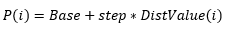(1)

where P(i) - parameter value, Base - base value of the parameter, step - scale coefficient (step) of change in the pseudorandom variable, DistValue(i) - generated pseudorandom variable distributed according to the specified law. Parameters Base and step are set by user. As an example, consider the code for forming value of the opening price:

```         if(i_bar>0)
MRatesMin[i_bar].open=MRatesMin[i_bar-1].close;
else
{
if((CopyClose(SName,PERIOD_M1,TCurrent-60,1,BValue)==-1))
MRatesMin[i_bar].open=NormalizeDouble(BaseOCHL+dOCHL*GetDist(DistOCHL,DistOCHLParam1,DistOCHLParam2,DistOCHLParam3),_Digits);
else
MRatesMin[i_bar].open=BValue;
}
```

If the bars are missing before the script is launched, or if the CopyClose function could not copy the last bar of the price history for some reason, the opening price of the first bar is formed according to the formula described above:

• BaseOCHL - base value for OCHL prices,
• dOCHL - scale coefficient of the OCHL price changes.

For subsequent bars, the Open price is equal to the previous Close price, i.e., a new bar opens at the Close of the previous one.

Generation of the pseudorandom variable's value is handled by the GetDist function, that takes the type of distribution and values of its parameters as input.

The IsErrCheck function is created to handle errors that occur during generation of the pseudorandom variable. As input, the function receives the error code determined during the execution of the GetDist function. If an error occurs, the execution of the script is interrupted, and an error message is printed to the log. The code of the GetDist and IsErrCheck functions is given at the end of the script.

The script generated minute ticks and has the following features:

1) Ticks are generated only in the specified time range, it is possible to not generate ticks on weekends (input parameter FiveDayOfWeek=true)

Disabling tick generation on weekends is implemented in the following code:

```if(FiveDayOfWeek)
{
TimeToStruct(TCurrent,StructCTime);
if(!((StructCTime.day_of_week!=0) && (StructCTime.day_of_week!=6)))
{
if(StructCTime.day_of_week==0)
TCurrent=TCurrent+86400-(StructCTime.hour*3600+StructCTime.min*60+StructCTime.sec);
else
TCurrent=TCurrent+2*86400-(StructCTime.hour*3600+StructCTime.min*60+StructCTime.sec);
if(TCurrent>=TEnd)
{
if(ReplaceBar==0)
Print("No trades in the specified range");
return;
}
}
}
```

If the current time falls on a weekend day, it is shifted to the next trading day.

2) The script allows replacing bars in parts, freeing memory after replacing the generated bars.

The number of bars, after which the array of generated ticks is zeroed, is specified in the BarForReplace variable. The replacement of bars is implemented in the following code:

```if(i_bar>=BarForReplace)
{
ReplaceHistory(MRatesMin.time,MRatesMin[i_bar-1].time);
i_bar=0;
ArrayFree(MRatesMin);
}
```

The ReplaceHistory function is created for replacing bars, its code is given at the end of the script. The bars are replaced by the CustomRatesReplace function. After replacing the bars, the counter is zeroed and the buffer of the MRatesMin dynamic array, that stores the created bars, is freed.

3) The script allows generating candles of different types

The generation of different types of candles is implemented as follows (parameter DiffCande = true):

``` if(DiffCandle)
{
i=MathRand()%5;
switch(i)
{
case 0:// Doji
{
MRatesMin[i_bar].close=MRatesMin[i_bar].open;
break;
}
case 1:// Hammer
{
if(MRatesMin[i_bar].open>MRatesMin[i_bar].close)
MRatesMin[i_bar].high=MRatesMin[i_bar].open;
else
{
if(MRatesMin[i_bar].open<MRatesMin[i_bar].close)
MRatesMin[i_bar].high=MRatesMin[i_bar].close;
}
break;
}
case 2:// Star
{
if(MRatesMin[i_bar].open>MRatesMin[i_bar].close)
MRatesMin[i_bar].low=MRatesMin[i_bar].close;
else
{
if(MRatesMin[i_bar].open<MRatesMin[i_bar].close)
MRatesMin[i_bar].low=MRatesMin[i_bar].open;
}
break;
}
case 3:// Maribozu
{
if(MRatesMin[i_bar].open>MRatesMin[i_bar].close)
{
MRatesMin[i_bar].high=MRatesMin[i_bar].open;
MRatesMin[i_bar].low=MRatesMin[i_bar].close;
}
else
{
if(MRatesMin[i_bar].open<MRatesMin[i_bar].close)
{
MRatesMin[i_bar].high=MRatesMin[i_bar].close;
MRatesMin[i_bar].low=MRatesMin[i_bar].open;
}
}
break;
}
default: break;
}
}
```

Thus, the script allows generating regular long or short candles, "Doji", "hammer", "star" and "maribozu" with the same probability.

Let us demonstrate the script operation. To do this, run the script with the following input parameters: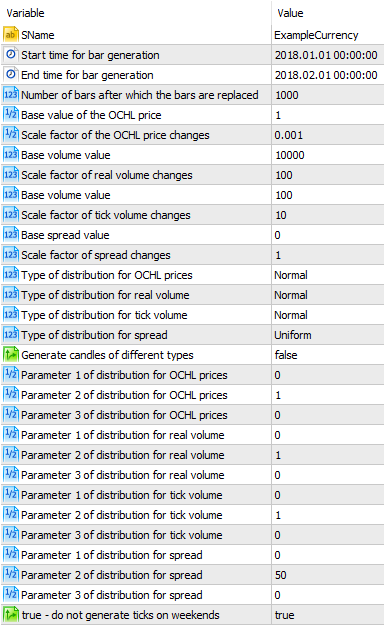Fig. 1. Input parameters of the script

As a result of the script execution, a new symbol, ExampleCurrency, will appear. 33121 minute bars were generated in the process of the script execution. Figure 2 shows a fragment of minute chart of the ExampleCurrency symbol.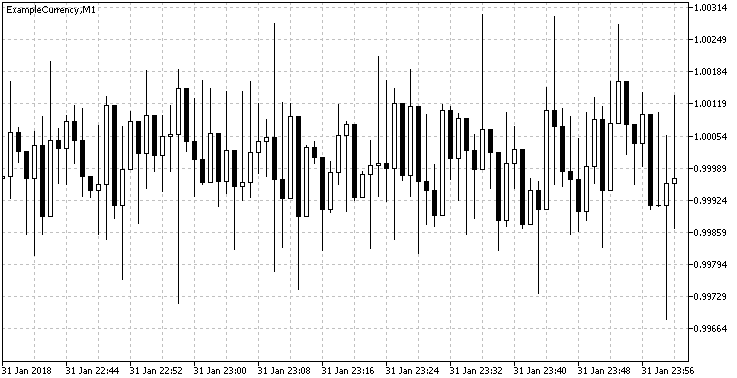Fig. 2. Minute chart of the ExampleCurrency symbol

Sometimes there may be insufficient minute bars for testing an Expert Advisor or an indicator, and testing is performed on real or simulated ticks.

Consider a script that simulates ticks and generated minute bars based on simulated ticks. The full code of the script is available in the GetTick.mq5 file attached to the article. Here is the code of the OnStart() function with descriptions:

```void OnStart()
{
if(SymbolInfoInteger(SName,SYMBOL_CUSTOM))// if the symbol exists
{
MqlDateTime  StructCTime;
long TBeginMSec=(long)TBegin*1000;      // start time for tick generation in ms
long TEndMSec=(long)TEnd*1000;          // end time for tick generation in ms
int ValMsec=0;                          // variable for generating a random time offset in ms
int SumSec=0;                           // seconds counter
int SumMSec=0;                          // milliseconds counter
int PrevTickCount=0;                    // variable for storing the previous number of ticks in a minute
datetime TCurrent=TBegin;
bool   NewMinute=false;
// copy the price value at which the tick generation starts
if(CopyClose(SName,PERIOD_M1,TCurrent-60,1,BValue)==-1)
BValue=Base;
// fill the LastTick structure
LastTick.bid=BValue;
LastTick.last=BValue;
LastTick.volume=baseVol;

while(TBeginMSec<=TEndMSec)
{
if(FiveDayOfWeek)
{
TimeToStruct(TCurrent,StructCTime);
if((StructCTime.day_of_week==0) || (StructCTime.day_of_week==6))
{
if(StructCTime.day_of_week==0)
{
TCurrent=TCurrent+86400;
TBeginMSec=TBeginMSec+86400000;
}
else
{
TCurrent=TCurrent+2*86400;
TBeginMSec=TBeginMSec+2*86400000;
}
if(TBeginMSec>=TEndMSec)
break;
}
}
GetTick(TCurrent,TBeginMSec);
if(IsErrCheck(DistErr)) return;
i_tick++;

if(RandomTickTime)
{
// generate a random time offset

ValMsec=(int)((MathRand()%30000)/(MaxTickInMinute*0.25)+1);
SumSec=SumSec+ValMsec;
SumMSec=SumMSec+ValMsec;
if(i_tick-PrevTickCount>=MaxTickInMinute)
{
TimeToStruct(TCurrent,StructCTime);
StructCTime.sec=0;
TCurrent=StructToTime(StructCTime)+60;
TBeginMSec=TBeginMSec+60000-SumSec+ValMsec;
SumSec=0;
SumMSec=0;
NewMinute=true;
}
else
{
if(SumSec>=60000)
{
// zero the counter of ticks per minute
SumSec=SumSec-60000*(SumSec/60000);
NewMinute=true;
}
// generate a new tick time
TBeginMSec=TBeginMSec+ValMsec;
if(SumMSec>=1000)
{
TCurrent=TCurrent+SumMSec/1000;
SumMSec=SumMSec-1000*(SumMSec/1000);
}
}
}
else
{
TBeginMSec=TBeginMSec+60000/MaxTickInMinute;
SumSec=SumSec+60000/MaxTickInMinute;
SumMSec=SumMSec+60000/MaxTickInMinute;
if(SumMSec>=1000)
{
TCurrent=TCurrent+SumMSec/1000;
SumMSec=SumMSec-1000*(SumMSec/1000);
}
if(SumSec>=60000)
{
SumSec=SumSec-60000*(SumSec/60000);
NewMinute=true;
}
}
if(NewMinute)
{
// add the new bar to the array
ArrayResize(MRatesMin,ArraySize(MRatesMin)+1);
if(ArraySize(MRatesMin)==1)// if it is the first minute
{
MRatesMin[i_bar].open=NormalizeDouble(LastTick.bid,_Digits);
MRatesMin[i_bar].tick_volume=(long)i_tick;
}
else
{
MRatesMin[i_bar].open=NormalizeDouble(MTick[PrevTickCount-1].bid,_Digits);
MRatesMin[i_bar].tick_volume=(long)i_tick-PrevTickCount;
}
MRatesMin[i_bar].close=NormalizeDouble(MTick[i_tick-1].bid,_Digits);
if(ValHigh>MRatesMin[i_bar].open)
MRatesMin[i_bar].high=NormalizeDouble(ValHigh,_Digits);
else
MRatesMin[i_bar].high=MRatesMin[i_bar].open;
if(ValLow<MRatesMin[i_bar].open)
MRatesMin[i_bar].low=NormalizeDouble(ValLow,_Digits);
else
MRatesMin[i_bar].low=MRatesMin[i_bar].open;
MRatesMin[i_bar].real_volume=(long)MTick[i_tick-1].volume;
TimeToStruct(MTick[i_tick-1].time,StructCTime);
StructCTime.sec=0;
MRatesMin[i_bar].time=StructToTime(StructCTime);
i_bar++;
PrevTickCount=i_tick;
ValHigh=2.2250738585072014e-308;
ValLow=1.7976931348623158e+308;
NewMinute=false;
if(i_bar>=BarForReplace)
{
ReplaceHistory(MRatesMin.time,MRatesMin[i_bar-1].time);
LastTick.bid=MTick[i_tick-1].bid;
LastTick.last=MTick[i_tick-1].last;
LastTick.volume=MTick[i_tick-1].volume;
i_tick=0;
i_bar=0;
PrevTickCount=0;
ArrayFree(MRatesMin);
ArrayFree(MTick);
}
}
}//end while
if(i_bar>0)
ReplaceHistory(MRatesMin.time,MRatesMin[i_bar-1].time);
}
else
Print("Symbol ",SName," does not exist");
}
```

The variables are initialized at the beginning of the OnStart() function. Generation of ticks and bars is performed in the main loop of the script:

```while(TBeginMSec<=TEndMSec)
{
...
}
```

At the beginning of the loop, if FiveDayOfWeek = true, tick generation on weekends is disabled, while the tick time is shifted by 1 day (if the tick time corresponds to Sunday) or 2 days (if the tick time corresponds to Saturday):

```if(FiveDayOfWeek)
{
...
}
```

Next, tick is generated using the GetTick function:

``` GetTick(TCurrent,TBeginMSec);
if(IsErrCheck(DistErr)) return;
i_tick++;
```

In case an error occurs (value of function IsErrCheck = true) during tick generation, the script execution will be interrupted. The IsErrCheck function is described above in the code of the GetCandle function.

Let us consider the GetTick function:

```void GetTick(datetime TDate,long TLong)
{
ArrayResize(MTick,ArraySize(MTick)+1);
// fill new time
MTick[i_tick].time=TDate;
MTick[i_tick].time_msc=TLong;
// fill the current tick with the values of the previous one
if(ArraySize(MTick)>1)
{
MTick[i_tick].bid=MTick[i_tick-1].bid;
MTick[i_tick].volume=MTick[i_tick-1].volume;
MTick[i_tick].last=MTick[i_tick-1].last;
}
else
{
MTick[i_tick].bid=LastTick.bid;
MTick[i_tick].last=LastTick.last;
MTick[i_tick].volume=LastTick.volume;
}
// fill the current tick
if(RandomTickValue)
{
double RBid=MathRandomUniform(0,1,DistErr);
double RVolume=MathRandomUniform(0,1,DistErr);
if(RBid>=0.5)
{
if(i_tick>0)
MTick[i_tick].bid=Base+GetDist(DistOCHL,DistOCHLParam1,DistOCHLParam2,DistOCHLParam3)*dStep;
MTick[i_tick].last=MTick[i_tick].bid;
MTick[i_tick].flags=10;
{
MTick[i_tick].flags=MTick[i_tick].flags+4;
}
if(RVolume>=0.5)
{
MTick[i_tick].volume=(ulong)(baseVol+GetDist(DistVolume,DistVolumeParam1,DistVolumeParam2,DistVolumeParam3)*dStepVol);
MTick[i_tick].flags=MTick[i_tick].flags+16;
}
}
else
{
{
MTick[i_tick].flags=4;
if(RVolume>=0.5)
{
MTick[i_tick].volume=(ulong)(baseVol+GetDist(DistVolume,DistVolumeParam1,DistVolumeParam2,DistVolumeParam3)*dStepVol);
MTick[i_tick].flags=MTick[i_tick].flags+16;
}
}
}
}//end if(RandomTickValue)
//+------------------------------------------------------------------+
//|                                                                  |
//+------------------------------------------------------------------+
else
{
MTick[i_tick].bid=Base+GetDist(DistOCHL,DistOCHLParam1,DistOCHLParam2,DistOCHLParam3)*dStep;
MTick[i_tick].last=MTick[i_tick].bid;
MTick[i_tick].volume=(ulong)(baseVol+GetDist(DistVolume,DistVolumeParam1,DistVolumeParam2,DistVolumeParam3)*dStepVol);
MTick[i_tick].flags=30;
}//end if(RandomTickValue)
// store the largest and the smallest values for generating minute bars
if(MTick[i_tick].bid>ValHigh)
ValHigh=MTick[i_tick].bid;
if(MTick[i_tick].bid<ValLow)
ValLow=MTick[i_tick].bid;
}//end
```

As input, the function takes the tick time in the datetime format and in milliseconds. First, the current tick is filled with the values of the previous tick, then the current tick values will be modified as follows:

1) If the RandomTickValue parameter value is true, each of the Ask, Bid and Volume parameters will be modified with a probability of 0.5. For this, 3 uniformly distributed random variables are generated:

```      double RBid=MathRandomUniform(0,1,DistErr);
double RVolume=MathRandomUniform(0,1,DistErr);
```

If RBid>0.5, RAsk>0.5 - Bid and/or Ask prices are modified according to the formula (1) described and/or for the GetCandle script. Modification of volume according to formula (1) is performed if the Ask or Bid price has changed and RVolume > 0.5. The Last price is assigned the Bid price value whenever it changes.

2) If RandomTickValue = false, values of parameters Ask, Bid and Volume are calculated according to formula (1).

The value of ticks flag (flags) is set as follows:

• change in the Bid price - flags=flags+2
• change in the Ask price - flags=flags+4
• change in the Last price - flags=flags+8
• change in the Volume - flags=flags+16

After the Ask, Bid or Volume price is modified, the maximum and minimum values of the Bid price are stored to variables ValHigh and ValLow. Value of the ValHigh and ValLow variables are used for generating the High and Low prices of the minute bar.

Let us continue considering the OnStart() function code.

Once the current tick is generated, the new tick appearance time is formed:

1) If the parameter RandomTickTime = true, the new tick time is formed in the following way:

`ValMsec=(int)((MathRand()%30000)/(MaxTickInMinute*0.25)+1);`

Next, the function checks the occurrence of a new minute bar, as well as adjusts the current time by the generated number of seconds. The number of ticks that can be generated during a single minute bar is limited by the MaxTickInMinute variable. If the number of the generated ticks exceeds the value of the MaxTickInMinute variable, the counters of seconds (SumSec) and milliseconds (SumMSec) are zeroed, and a new minute bar is formed (NewMinute = true).

2) If the parameter RandomTickTime = false, then the same number of ticks specified in the MaxTickInMinute variable is generated in each minute bar.

The minute bar based on the generated ticks is performed as follows:

• the array of minute bars is increased by 1

` ArrayResize(MRatesMin,ArraySize(MRatesMin)+1);`

• a new Open price value and tick volume of the current bar is generated:

```            if(ArraySize(MRatesMin)==1)// if it is the first minute
{
MRatesMin[i_bar].open=NormalizeDouble(LastTick.bid,_Digits);
MRatesMin[i_bar].tick_volume=(long)i_tick;
}
else
{
MRatesMin[i_bar].open=NormalizeDouble(MTick[PrevTickCount-1].bid,_Digits);
MRatesMin[i_bar].tick_volume=(long)i_tick-PrevTickCount;
}
```

When forming the first minute bar, the Open price is assigned the Bid price of the previous tick from the previous tick replacement (replacement of ticks and bars is performed by the ReplaceHistory function) or the base value of the Bid price (parameter Base), in case the replacement of bars and ticks has not been performed yet. When forming the subsequent minute bars, the Open price is assigned the normalized value of the Bid price from the last tick (Close price) of the previous minute. Normalization is regarded to as rounding to the measurement precision of the symbol on the current chart the script is running on.

• Close price value is formed - the normalized Bid price of the last tick:

`MRatesMin[i_bar].close=NormalizeDouble(MTick[i_tick-1].bid,_Digits);`

• High and Low price values are formed. This takes into account that the Open price value may be greater than the highest (ValHigh) or less than the smallest (ValLow) Bid price value of the generated ticks:

``` if(ValHigh>MRatesMin[i_bar].open)
MRatesMin[i_bar].high=NormalizeDouble(ValHigh,_Digits);
else
MRatesMin[i_bar].high=MRatesMin[i_bar].open;
if(ValLow<MRatesMin[i_bar].open)
MRatesMin[i_bar].low=NormalizeDouble(ValLow,_Digits);
else
MRatesMin[i_bar].low=MRatesMin[i_bar].open;
```

• value and spread values are formed:
```MRatesMin[i_bar].real_volume=(long)MTick[i_tick-1].volume;
```

The volume of the current bar is assigned the value of the last tick's volume, spread is calculated as the difference between the Bid and Ask prices of the last tick.

• bar opening time is assigned the last tick's creation time value with zeroed seconds value:
```TimeToStruct(MTick[i_tick-1].time,StructCTime);
StructCTime.sec=0;
MRatesMin[i_bar].time=StructToTime(StructCTime);
```

This concludes the process of forming the parameters of the current minute bar, the counter of minute bars (variable i_bar) is increased, variables ValHigh and ValLow are assigned the minimum and the maximum values of the double data type, the minute bar flag (NewMinute) is reset. Next, it is checked if it is time to replace the formed number of minute bars and ticks. The number of bars, after forming which they are replaced, is set in the BarForReplace variable.

After exiting the main loop, the remaining bars are replaced, if any:

```     if(i_bar>0)
ReplaceHistory(MRatesMin.time,MRatesMin[i_bar-1].time);
```

The replacement of ticks and bars is implemented in the ReplaceHistory function. Ticks are replaced by the CustomTicksReplace function, bars are replaced by the CustomRatesReplace function.

Thus, the GetTick script described above allows generating ticks according to the specified distribution laws, and to use the ticks to form minute bars and to load the generated data into the price history of the custom symbol.

### Simulating the trend

The GetCandle and GetTick scripts considered in the previous section allow generating the price history without strong price fluctuations, i.e., in the flat. To form more complex market situations and testing Expert Advisors and indicator using them, it is necessary to simulate a trend price movement.

For these purposes, the scripts GetCandleTrend (attached to the article in the file GetCandleTrend.mq5) and GetTickTrend (attached to the article in the file GetTickTrend.mq5) have been created. They allow simulating ascending and descending trends according to a given law of price movement. The GetCandleTrend script is designed to generate increasing or decreasing minute bars. The GetTickTrend script generates increasing or decreasing ticks, then forms minute bars, similarly to the GetCandleTrend script.

Let us consider the operation of the GetCandleTrend script. The generation of minute bars is similar to that in the GetCandle script, therefore, only the trend generation method will be considered. The script input data contain the following parameters of the trend:

```input TrendModel TModel = Linear;                // Trend model
input TrendType TType =  Increasing;             // Trend type (increasing/decreasing, random)
input double RandomTrendCoeff=0.5;               // Trend coefficient (if RandomTrendCoeff<0.5 the descending trend is dominant; if RandomTrendCoeff>0.5 - ascending)
input double Coeff1=0.1;                         // The k1 coefficient of the trend model
input double Coeff2=0.1;                         // The k2 coefficient of the trend model
input double Coeff3=0.1;                         // The k3 coefficient of the trend model
input int CountCandle=60;                        // Interval of random change in the trend direction (in bars)
```

The user is prompted to select the trend model set in the TrendModel enumeration:

```enum TrendModel
{
Linear,
Hyperbolic,
Exp,
Power,
SecondOrderPolynomial,
LinearAndPeriodic,
LinearAndStochastic
};
```

and the trend type set in the TrendType enumeration:

```enum TrendType
{
Increasing,
Decreasing,
Random
};
```

The trend is formed according to the following models: linear, hyperbolic, exponential, power, parabolic, linear periodic and linear stochastic. The formulas for generating the trend are listed in table 1:

Trend modelFormula
Linear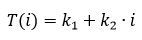Hyperbolic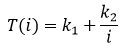Exponential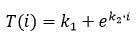Power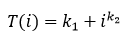Parabolic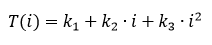Linear periodic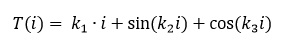Linear stochastic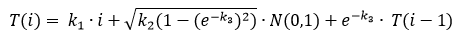T(i) - the current trend value; k1, k2, k3 - coefficients affecting the rate of trend increase (decrease); N(0,1) - random variable distributed according to the normal law with expected value of zero and unit variance.

As the trend type, the user can select increasing, decreasing or random trend. A random trend is a trend with its direction changing after a given number of candles (the number of candles is set in the Countcandle parameter).

The trend formation is implemented in the ChooseTrend function:

```double ChooseTrend()
{
switch(TType)
{
case 0: return NormalizeDouble(BValue+dOCHL*(GetTrend()+GetDist(DistOCHL,DistOCHLParam1,DistOCHLParam2,DistOCHLParam3)),_Digits);
case 1: return NormalizeDouble(BValue+dOCHL*(-GetTrend()+GetDist(DistOCHL,DistOCHLParam1,DistOCHLParam2,DistOCHLParam3)),_Digits);
case 2:
{
if((i_trend%CountCandle==0) && (i_trend!=0))
{
if(i_bar!=0)
BValue=MRatesMin[i_bar-1].close;
LastRand=MathRandomUniform(0,1,DistErr);
i_trend=0;
}
if(LastRand>RandomTrendCoeff)
return NormalizeDouble(BValue+dOCHL*(GetModelTrend()+GetDist(DistOCHL,DistOCHLParam1,DistOCHLParam2,DistOCHLParam3)),_Digits);
else
return NormalizeDouble(BValue+dOCHL*(-GetModelTrend()+GetDist(DistOCHL,DistOCHLParam1,DistOCHLParam2,DistOCHLParam3)),_Digits);
}
default:return 0;
}
}
```

In case of a random trend, for every N minute candles set in the CountCandle parameter, a random variable LastRand is generated, uniformly distributed in the range from 0 to 1. If the LastRand value is greater than the RandomTrendCoeff parameter, the trend is ascending, otherwise — descending. The RandomTrendCoeff probability allows varying the probability of trend changes. If RandomTrendCoeff<0.5 the ascending trend will be prevalent, if RandomTrendCoeff>0.5 — descending.

Generation of a trend with the specified model is implemented in the GetModelTrend function:

```double GetModelTrend()
{
switch(TModel)
{
case Linear: return Coeff1+Coeff2*i_trend;
case Hyperbolic:
{
if(i_trend==0)
return Coeff1;
else
return Coeff1+Coeff2/i_trend;
}
case Exp:
{
if(i_trend==0)
return Coeff1;
else
return Coeff1+MathExp(Coeff2*i_trend);
}
case Power:return Coeff1+MathPow((double)i_trend,Coeff2);
case SecondOrderPolynomial:return Coeff1+Coeff2*i_trend+Coeff3*i_trend*i_trend;
case LinearAndPeriodic: return Coeff1*i_trend+sin(Coeff2*i_trend)+cos(Coeff3*i_trend);
case LinearAndStochastic:
{
LastValue=Coeff1*i_trend+MathSqrt(Coeff2*(1-MathPow(exp(-Coeff3),2)))*MathRandomNormal(0,1,DistErr)+exp(-Coeff3)*LastValue;
return LastValue;
}
default:
return -1;
}
}
```

The trend models shown in table 1 are implemented in this function.

Let us consider the generation of price charts for different trend models. Generate a linear trend by running the GetTrendCandle script with the following parameters: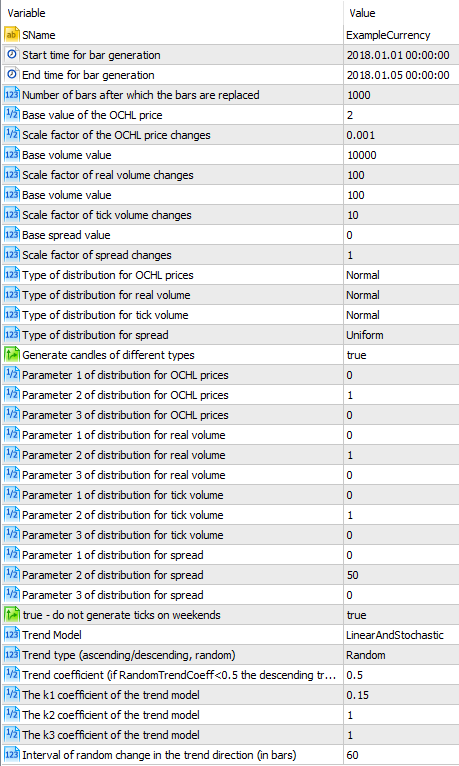Fig. 3. Parameters of the GetTrendCandle script

After the script has been executed, open the minute chart of the ExampleCurrency symbol: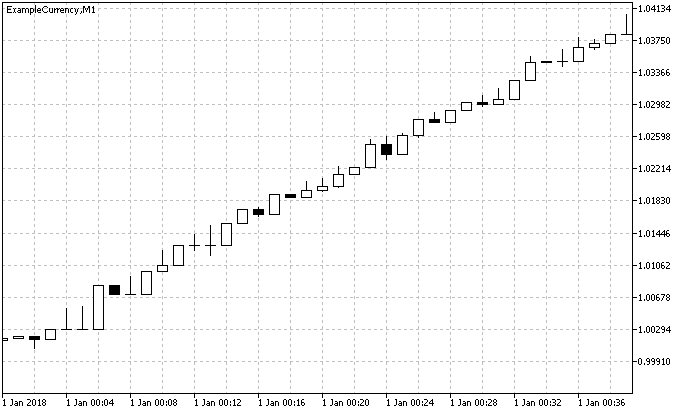Fig. 4. Minute chart of the ExampleCurrency symbol for the linear trend model

It can be seen from the chart that a linear trend was formed, the trend inclination angle (rate of increase/decrease) can be changed by varying the coefficients k1 and k2.

Specify the hyperbolic trend model in the script parameters: TModel = Hyperbolic, Coeff1 = 1, Coeff2 = 1000. After running the script, the following chart is obtained: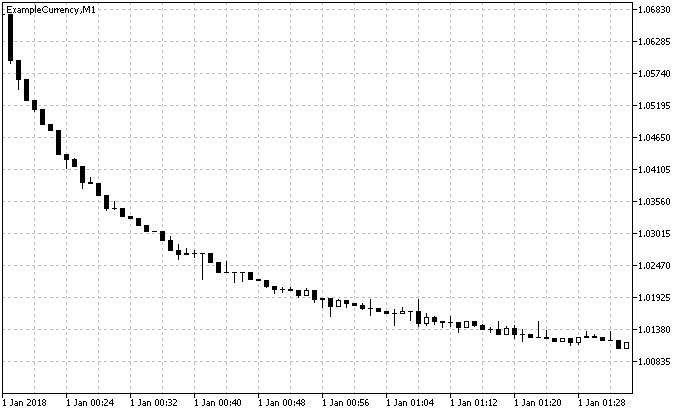Fig. 4. Minute chart of the ExampleCurrency symbol for the hyperbolic trend model

This model has these features: due to the fact that the hyperbolic function belongs to the class of inverse functions, the trend will be descending when choosing an ascending trend (TType = Increasing).

Let us consider the exponential trend model: TModel =Exp, Coeff1 = 1, Coeff2 = 0,1. After running the script, the following chart is obtained: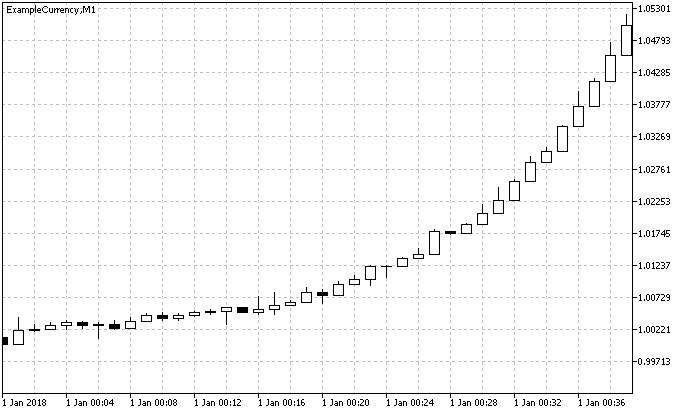Fig. 5. Minute chart of the ExampleCurrency symbol for the exponential trend model

As one would expect, the graph shows an exponentially increasing trend with an increasing size of candles.

Consider other trend models:

Power trend model: TModel =Power, Coeff1 = 1, Coeff2 = 2.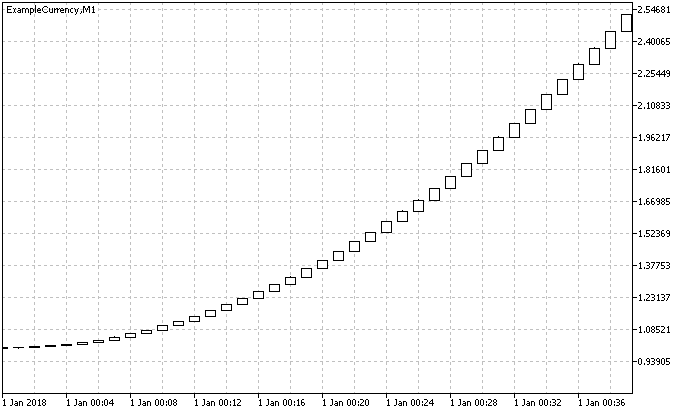Fig. 6. Minute chart of the ExampleCurrency symbol for the power trend model

It can be seen that the chart is similar to the exponential trend model, but increases more smoothly.

Parabolic trend model: TModel = SecondOrderPolynomial, Coeff1 = 1, Coeff2 = 0,05, Coeff3 = 0,05.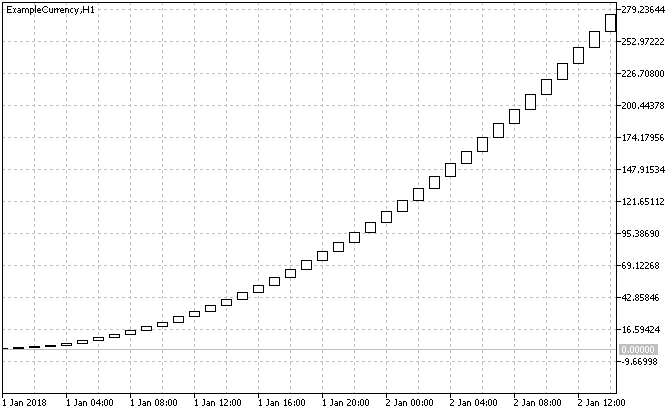Fig. 7. Minute chart of the ExampleCurrency symbol for the parabolic trend model

The parabolic model is similar to the power model, but its trend increases/decreases at a higher rate.

Linear periodic trend model: TModel = LinearAndPeriodic, Coeff1 = 0.05, Coeff2 = 0,1, Coeff3 = 0,1.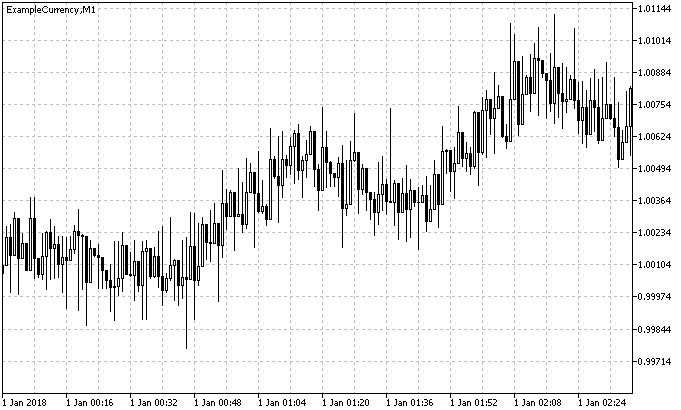Fig. 8. Minute chart of the ExampleCurrency symbol for the linear parabolic trend model

In the linear periodic model, as the trend increases or decreases, it changes its direction according to the periodic law.

Linear stochastic trend model: TModel = LinearAndStochastic, Coeff1 = 0.05, Coeff2 = 1, Coeff3 = 1.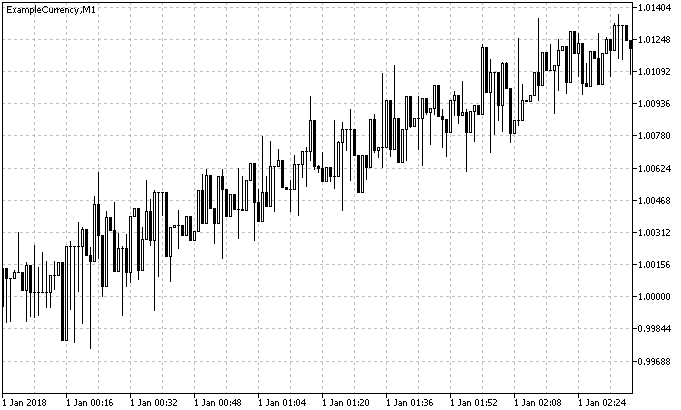Fig. 8. Minute chart of the ExampleCurrency symbol for the linear stochastic trend model

In the linear stochastic model, the trend increases or decreases by making random fluctuations about a straight line, the inclination of which is defined by the k1 coefficient.

The trend models discussed above have the specified properties only on minute timeframes. The price charts generated for these models look like linear functions on timeframes M15, M30, H1 and higher. In order to obtain a trend that changes its direction on timeframes other than M1, it is necessary to select the random trend type (TType = Random) and to specify the number of minute candles after which to make an attempt to change the trend direction.

Run the script with the following parameters: TModel = LinearAndStochastic, TType =  Random, Coeff1 = 0.05, Coeff2 = 1, Coeff3 = 1, RandomTrendCoeff=0.5, CountCandle=60. The following chart on the H1 timeframe is obtained: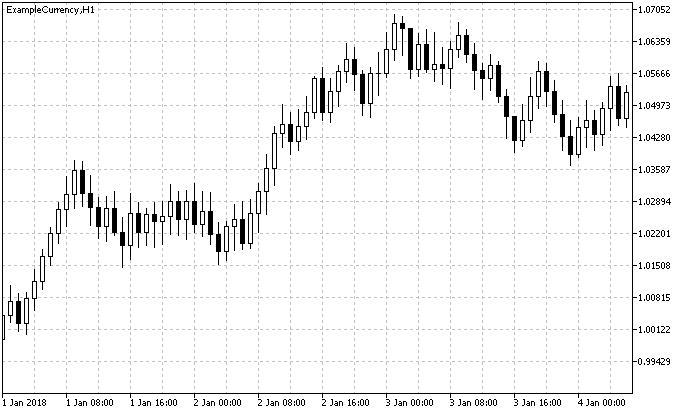Fig. 9. Hourly chart of the ExampleCurrency symbol with random trend change

Set the parameter RandomTrendCoeff = 0.7 and run the script: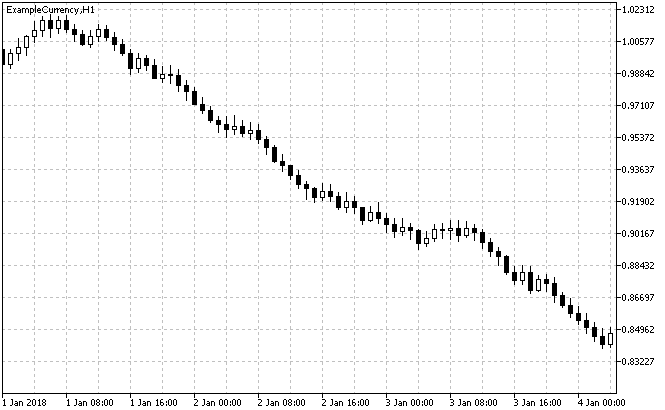Fig. 10. Hourly chart of the ExampleCurrency symbol with random trend change with RandomTrendCoeff = 0.7

As you can see, a descending trend is present, change RandomTrendCoeff to 0.3 and get an ascending trend: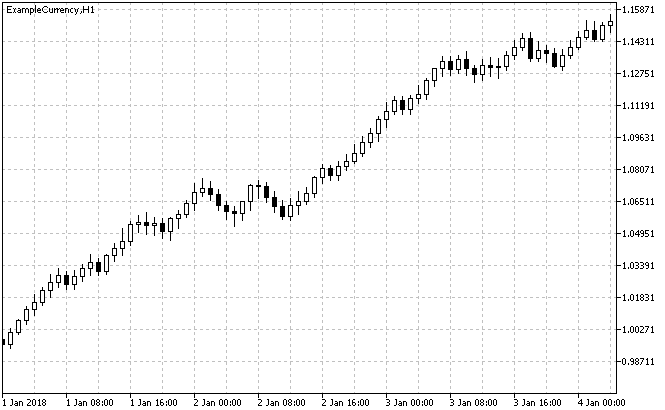Fig. 10. Hourly chart of the ExampleCurrency symbol with random trend change with RandomTrendCoeff = 0.3

Thus, it is possible to simulate a trend at higher timeframes with the GetCandleTrend script, while generating minute bars.

The GetTickTrend script allows generating ticks and to use them for forming minute bars. It also has the same capabilities as the GetCandleTrend script.

### Simulating chart patterns

Chart patterns are widely used in technical analysis of the market. Many traders use typical patterns to search for market entry or exit points. Also, various indicators and Experts are developed for analyzing patterns on the price chart.

In this section, we will show how to create chart patterns using the scripts described above. As an example, consider the process of creating the "Double Top" and "Double Bottom" patterns. The appearance of the patterns is shown in figures below: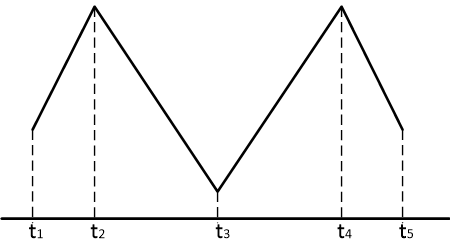Fig. 11. "Double Top" pattern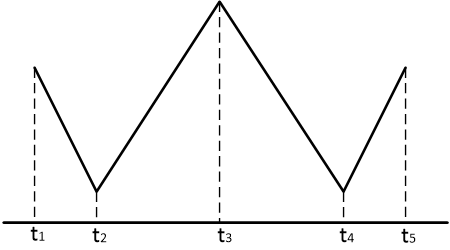Fig. 12. "Double Bottom" pattern

The GetCandleTrend script will be used for creating patterns. The patterns will be formed on the H1 period. To form each pattern, it is necessary to run the GetCandleTrend script four times with different input parameters. Select the following time intervals, indicated in figures 11 and 12 as t1-t5:

• t1 - 00:00 02.01.2018
• t2 - 13:00 02.01.2018
• t3 - 13:00 03.01.2018
• t4 - 13:00 04.01.2018
• t5 - 00:00 05.01.2018

Set the following script settings to generate the "Double Top" pattern:

• Bar generation start time: 00:00 02.01.2018
• Bar generation end time: 12:00 02.01.2018
• Trend model: LinearAndStochastic
• Trend type: Random
• Trend coefficient: 0.15
• Coefficient k1 of the trend model: 0.15
• Coefficient k2 of the trend model: 1
• Coefficient k3 of the trend model: 1
• Interval of random change in the trend direction: 60
Leave the default values in the remaining parameters and run the script. Next, for the second, third and fourth run of the script, change the following settings:

Run #2:

• Bar generation start time: 13:00 02.01.2018
• Bar generation end time: 12:00 03.01.2018
• Trend coefficient: 0.85
Run #3:

• Bar generation start time: 13:00 03.01.2018
• Bar generation end time: 12:00 04.01.2018
• Trend coefficient: 0.15
Run #4:

• Bar generation start time: 13:00 04.01.2018
• Bar generation end time: 00:00 05.01.2018
• Trend coefficient: 0.85

As a result, after running the GetCandleTrend script for four times, the price chart shown in figure 13 will be obtained.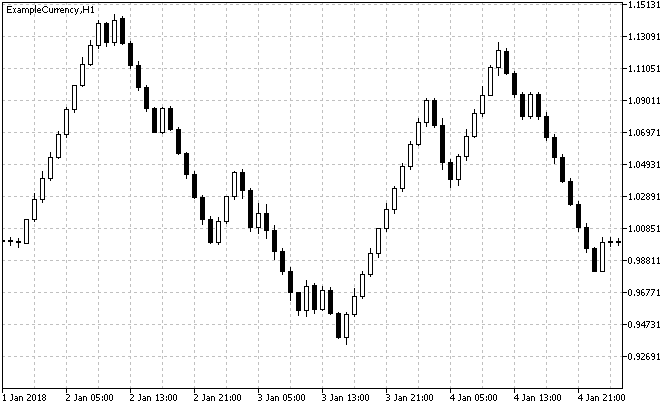Fig. 13. Simulated "Double Top" pattern on the H1 period

The "Double Bottom" pattern is simulated similarly. To do this, run the GetCandleTrend script four times with the settings specified for the "Double Top" pattern, changing only the trend factor: 0.85 for the first run, 0.15, 0.85, 0.15 for the next ones. The result of the script is shown in Figure 14.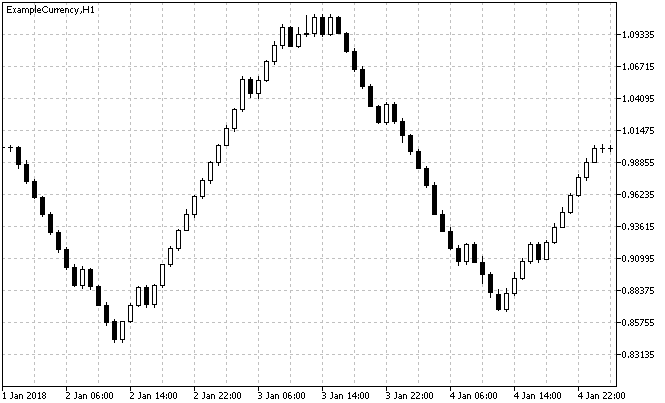Fig. 14. Simulated "Double Bottom" pattern on the H1 period

Similarly, it is possible to simulate other patterns. The most realistic patterns are obtained on a minute chart. To form patterns on other timeframes, it is necessary to specify the number of minute candles contained in the selected timeframe in the parameter "Interval of random change in the trend direction" of the GetCandleTrend script. For example, for the H1 timeframe - 60, for the H4 timeframe - 240.

### Conclusion

Custom symbols are a convenient and useful tool for testing experts and indicators. In this article, scripts with the following features were created and considered:

1) Creating and removing custom symbols

Methods for creating a custom symbol based on an existing or a new symbol with the manually specified properties are shown. The script that implements the removal of a symbol allows closing all charts with the symbol and to remove it from the Market Watch.

2) Generating ticks and bars

The scripts allow generating minute bars in the specified time interval, with the ability to generate bars on weekend (non-trading) days. Generation of different candles is implemented: long or short candles, "doji", "hammer", "star" and "maribozu". Replacement of bars and ticks in parts is also implemented to save memory when generating large arrays of bars and ticks.

3) Simulating the trend

The scripts allow simulating the trend according to different models: linear, hyperbolic, exponential, power, parabolic, linear periodic, and linear stochastic. It is also possible to simulate a trend on different periods.

4) Simulating chart patterns

An example shows the use of the GetCandleTrend script for creating the "Double Top" and "Double Bottom" patterns.

The proposed scripts can be used for creating a custom price history from minute bars and ticks, testing and optimizing experts and indicators.

Translated from Russian by MetaQuotes Software Corp.
Original article: https://www.mql5.com/ru/articles/4566

Attached files |
CreateSymbol.mq5 (28.79 KB)
DeleteSymbol.mq5 (6.96 KB)
GetCandle.mq5 (33.06 KB)
GetCandleTrend.mq5 (40.66 KB)
GetTick.mq5 (39.14 KB)
GetTickTrend.mq5 (46.32 KB)
Last comments | Go to discussion (4)| 31 Oct 2018 at 06:37
Would like an article on creating json files for custom symbols.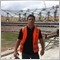| 28 Jan 2019 at 15:35
Incredible article, I needed to learn the symbol creation code customized by EA, now it's easy with the help of your article, it was even easy to understand how the symbol creation code is written. thank you very much. Bright article.

Unfortunately the tick creation part is too complex for my programming ability, but over time I learn.

Just do not understand why MT5 erases custom symbols every time it reboots, would you know the reason or how to solve?| 9 Mar 2019 at 08:54
Жоао Луис Са Марчиоро :
Невероятная статья, мне нужно было изучить код создания символа, настроенный EA, теперь легко понять, как пишется код создания символа. большое спасибо вам. Яркая статья.

К сожалению, я не могу учиться.

Просто не понимаю, почему MT5 стирает пользовательские символы каждый раз при перезагрузке,

Я не сталкивался с такой проблемой. Все созданные пользовательские символы и открытые графики сохраняются после перезагрузки MT5. Возможно, вам стоит обновить вашу версию MT5.| 9 Mar 2019 at 09:07
Aleksey Zinovik:

Я не сталкивался с такой проблемой. Все созданные пользовательские символы и открытые графики сохраняются после перезагрузки MT5. Возможно, вам стоит обновить вашу версию MT5.

This is an English language forum.

Use the translation tool if necessary.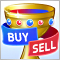Reversing: The holy grail or a dangerous delusion?

In this article, we will study the reverse martingale technique and will try to understand whether it is worth using, as well as whether it can help improve your trading strategy. We will create an Expert Advisor to operate on historic data and to check what indicators are best suitable for the reversing technique. We will also check whether it can be used without any indicator as an independent trading system. In addition, we will check if reversing can turn a loss-making trading system into a profitable one.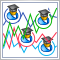Using indicators for optimizing Expert Advisors in real time

Efficiency of any trading robot depends on the correct selection of its parameters (optimization). However, parameters that are considered optimal for one time interval may not retain their effectiveness in another period of trading history. Besides, EAs showing profit during tests turn out to be loss-making in real time. The issue of continuous optimization comes to the fore here. When facing plenty of routine work, humans always look for ways to automate it. In this article, I propose a non-standard approach to solving this issue.100 best optimization passes (part 1). Developing optimization analyzer

The article dwells on the development of an application for selecting the best optimization passes using several possible options. The application is able to sort out the optimization results by a variety of factors. Optimization passes are always written to a database, therefore you can always select new robot parameters without re-optimization. Besides, you are able to see all optimization passes on a single chart, calculate parametric VaR ratios and build the graph of the normal distribution of passes and trading results of a certain ratio set. Besides, the graphs of some calculated ratios are built dynamically beginning with the optimization start (or from a selected date to another selected date).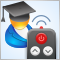EA remote control methods

The main advantage of trading robots lies in the ability to work 24 hours a day on a remote VPS server. But sometimes it is necessary to intervene in their work, while there may be no direct access to the server. Is it possible to manage EAs remotely? The article proposes one of the options for controlling EAs via external commands.